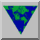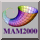# Math Rules

A segment is a one-dimensional object. Once we know the location of the endpoints, we can specify each point on the segment by using just one number, going from 0 at one endpoint to 1 at the other. The midpoint is specified by 1/2, the point halfway between the midpoint and the end by 3/4, and so on.

To locate a segment, we have to tell where its endpoints are, and the number of coordinates for each point can be large. If we want a segment that joins a point on one coordinate axis to a point on another, then we need two numbers. If we want a segment with starting point on one line and ending point in a given plane, then we need three numbers. The collection of all segments in the plane is four-dimensional since we need two coordinates for each of the endpoints. So the location of a segment can be specified using any number of numbers, even though the segment itself is one-dimensional.

An example of a one-dimensional application is a core sample taken by a geologist, boring a deep shaft into the earth and then analyzing what lies at each depth. Each observation can be specified by a single number, how far down the shaft is lies. Of course it is necessary to tell the location of the shaft, and that takes at least two more numbers, latitude and longitude. For a discussion of this application select Thompson Webb, Paleoecology.

A circle is one-dimensional, in the sense that it only takes one number to give the location of a point on the circle, once we have a starting point and a direction of travel along the circle. Traveling the length 2pR along a circle of radius R brings us back to the starting point. Traveling pR along the circle brings us halfway around, and in order to get 3/4 of the way around, it is necessary to go a distance of (3/4)(2pR). Another way of specifying a point on a circle is to give its angle, so that each point is a certain number of degrees from the origin, anywhere from 0 to 360. We can also use a clock to specify a point on the circumference by the position of the minute hand, from noon to one p.m.

The position of a car racing along an oval race track can be given by a single number, the distance from the starting point. This is also true for race tracks with more complicated shapes. No matter what the shape of the race course is, it is essentially one- dimensional. If we know the length of the track and we know how far the care has traveled, then we can figure out where it is on the track. Of course there are many other things we would want to know about a race car besides its position, for example its velocity and its acceleration. These have to do with the way the car traverses the track and there are infinitely many ways to do this, even though the track itself is one- dimensional. It is also true that a track is wide as well as long, a crucial feature if one car is going to be able to pass another, but the most important dimension is length. To see more about the mathematics of car racing, select the entry for Richard Tapia.

One-dimensional objects have length. A segment has length, and so does a circle (its circumference). A ray or an entire straight line will have infinite length. Even though each circle is one-dimensional as a mathematical object, it may take many numbers to specify a particular circle. For example a circle in the plane can be specified by the location of its center and the length of its radius. We can say that the collection of circles in the plane is a three-dimensional collection of one-dimensional objects, just as the collection of segments in the plane is a four-dimensional collection of one-dimensional objects.Math Awareness Month 2000 Last modified: 28 Mar 2000 14:05:11 Comments to: `thomas_banchoff@brown.edu` Hosted by: The Math Forum